# RS Aggarwal Solutions for Class 9 Chapter 18: Mean, Median and Mode of Ungrouped Data Exercise 18C

## RS Aggarwal Solutions for Class 9 Maths Exercise 18C PDF

RS Aggarwal Solutions can be used by students as a reference guide to prepare well for the exams. The exercise wise solutions are prepared by an expert team of faculty having experience in the education industry. By using the solutions, students can analyse the areas of weaknesses and improve on them. The exercise wise solutions which are explained in simple language help in gaining conceptual knowledge. The PDF is available for free which can be downloaded by the students to boost their exam preparation. RS Aggarwal Solutions for Class 9 Maths Chapter 18 Mean, Median and Mode of Ungrouped Data Exercise 18C are provided here.

## RS Aggarwal Solutions for Class 9 Chapter 18: Mean, Median and Mode of Ungrouped Data Exercise 18C Download PDF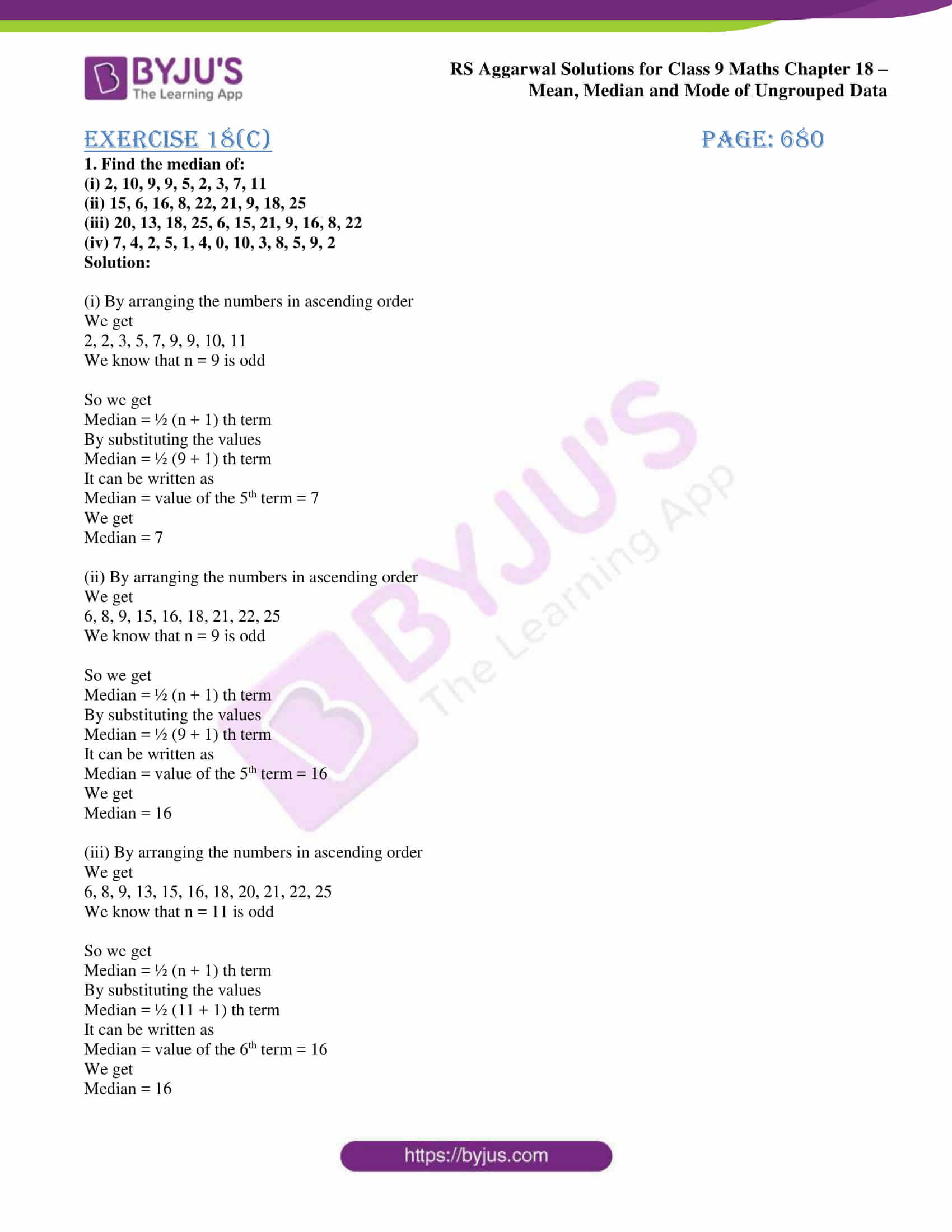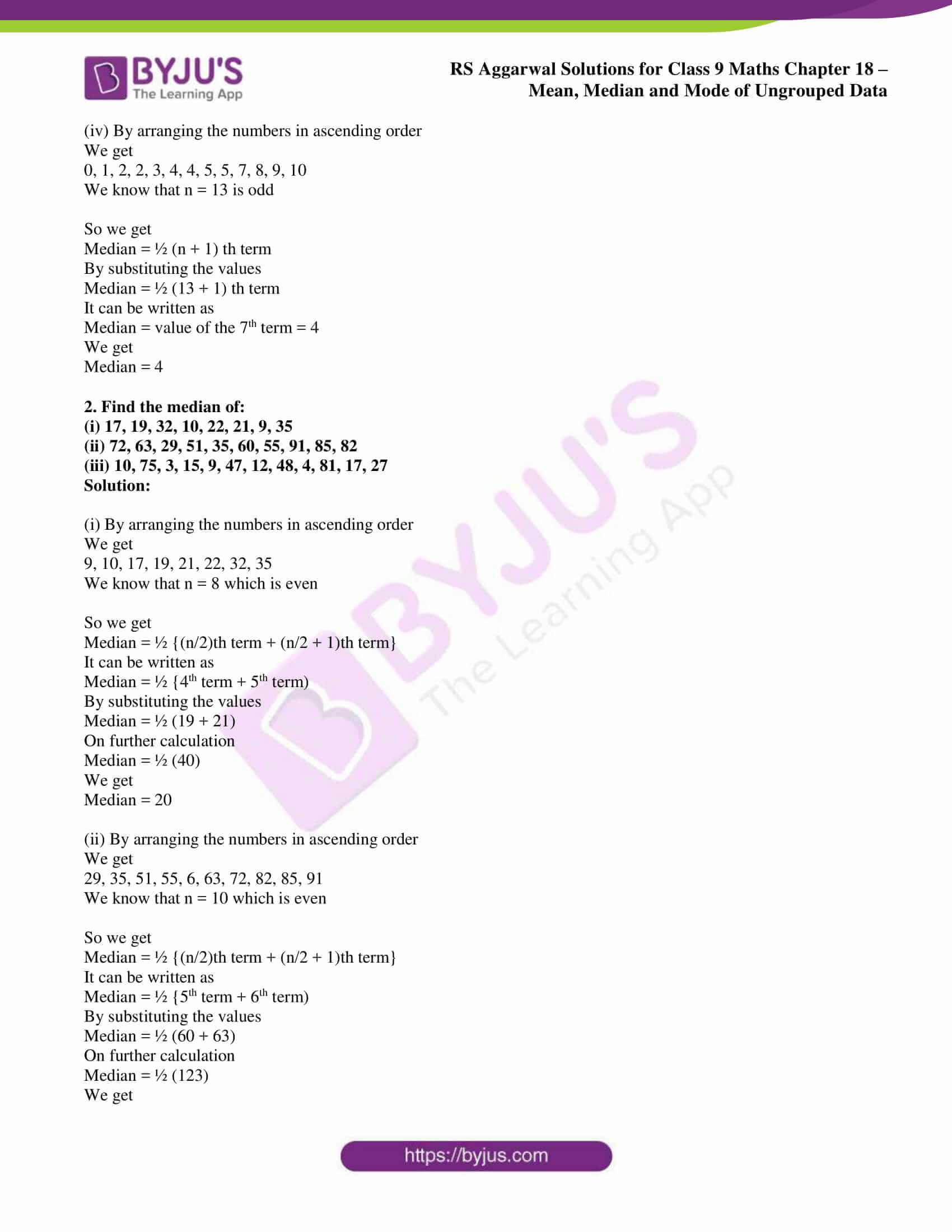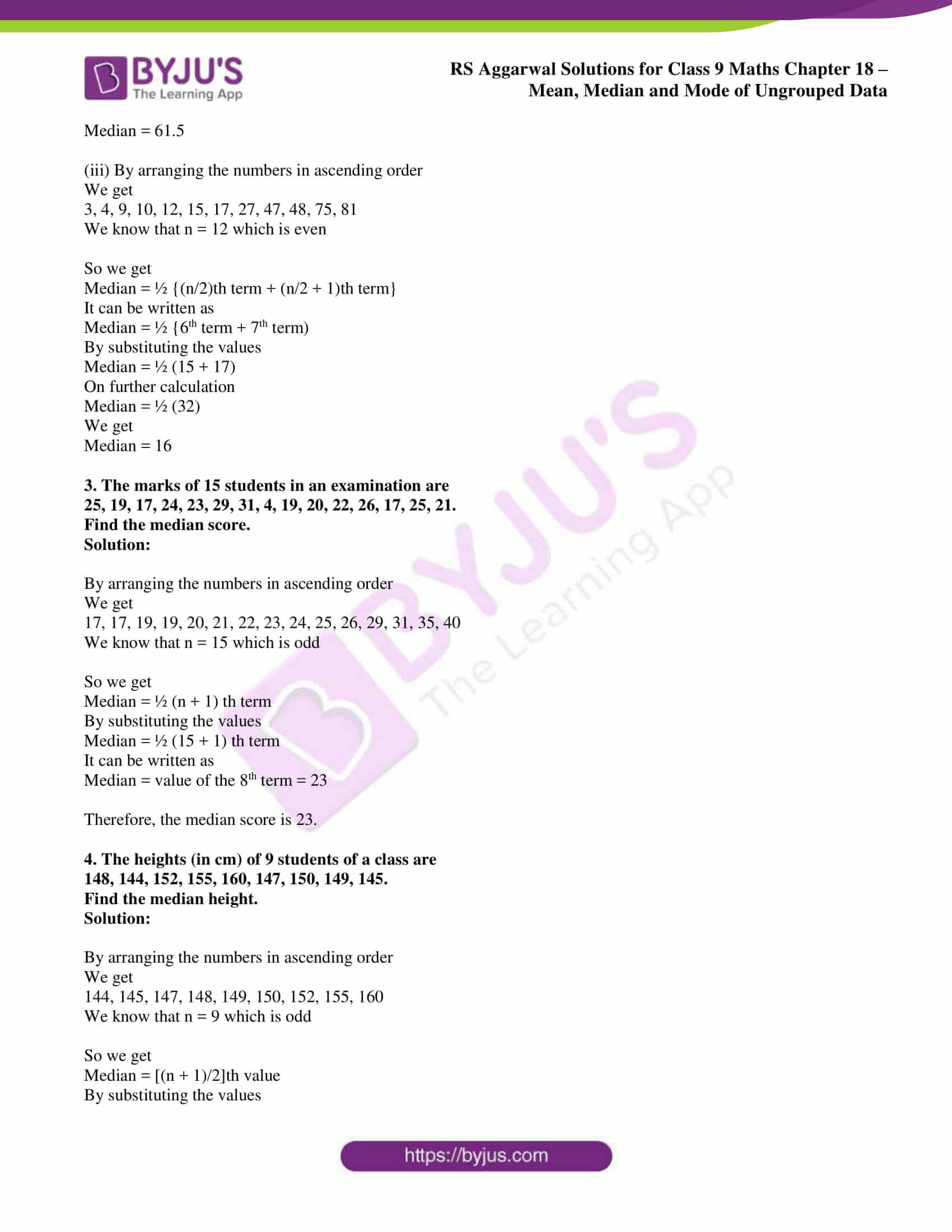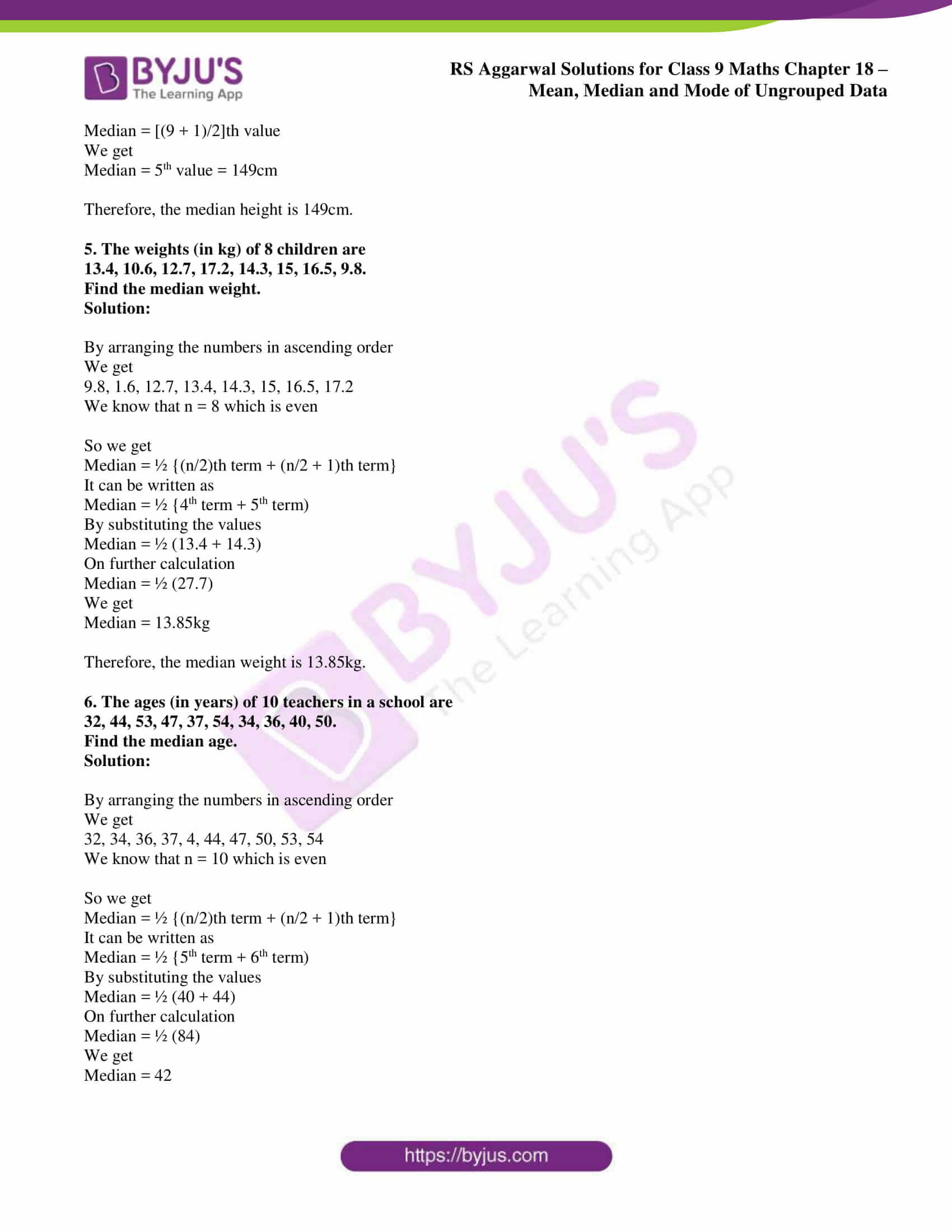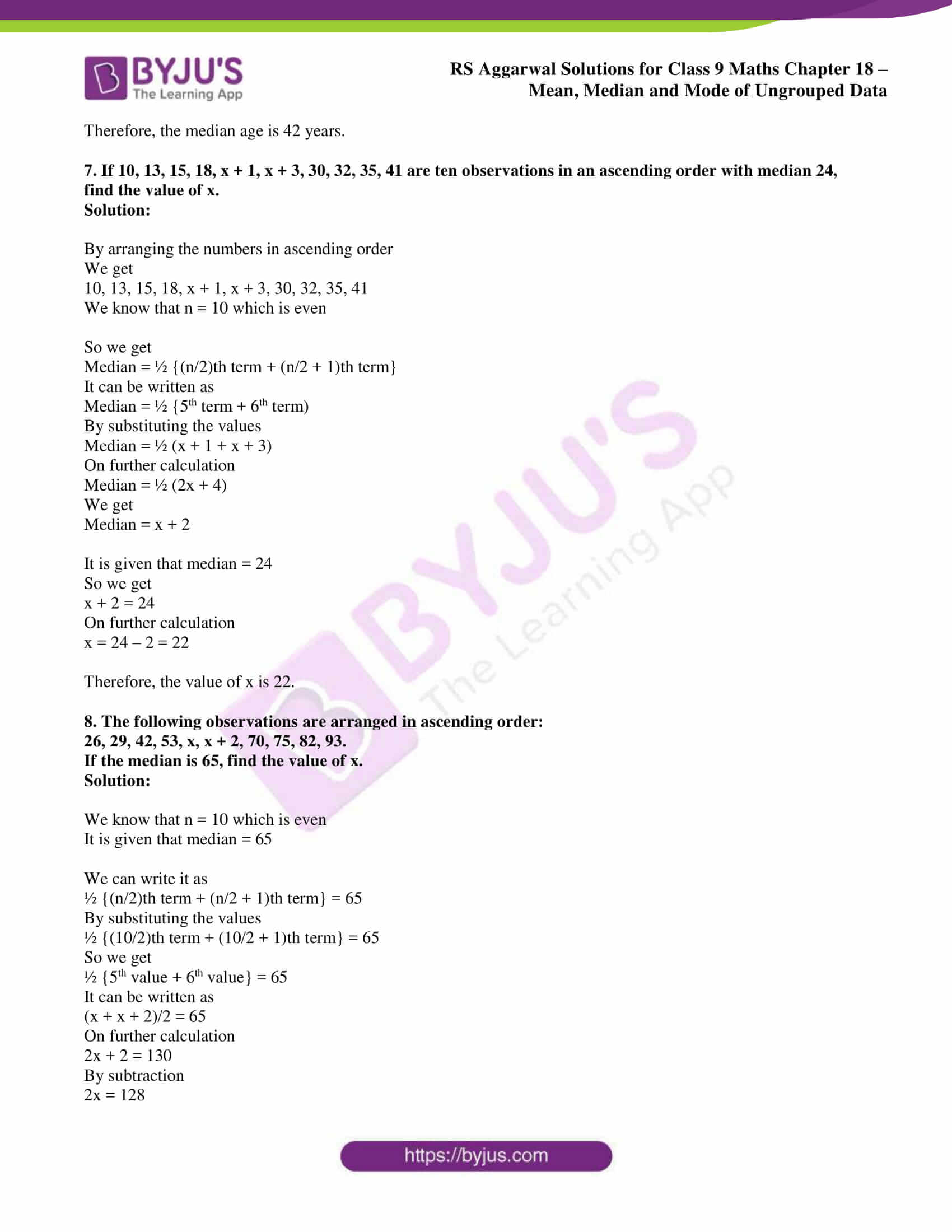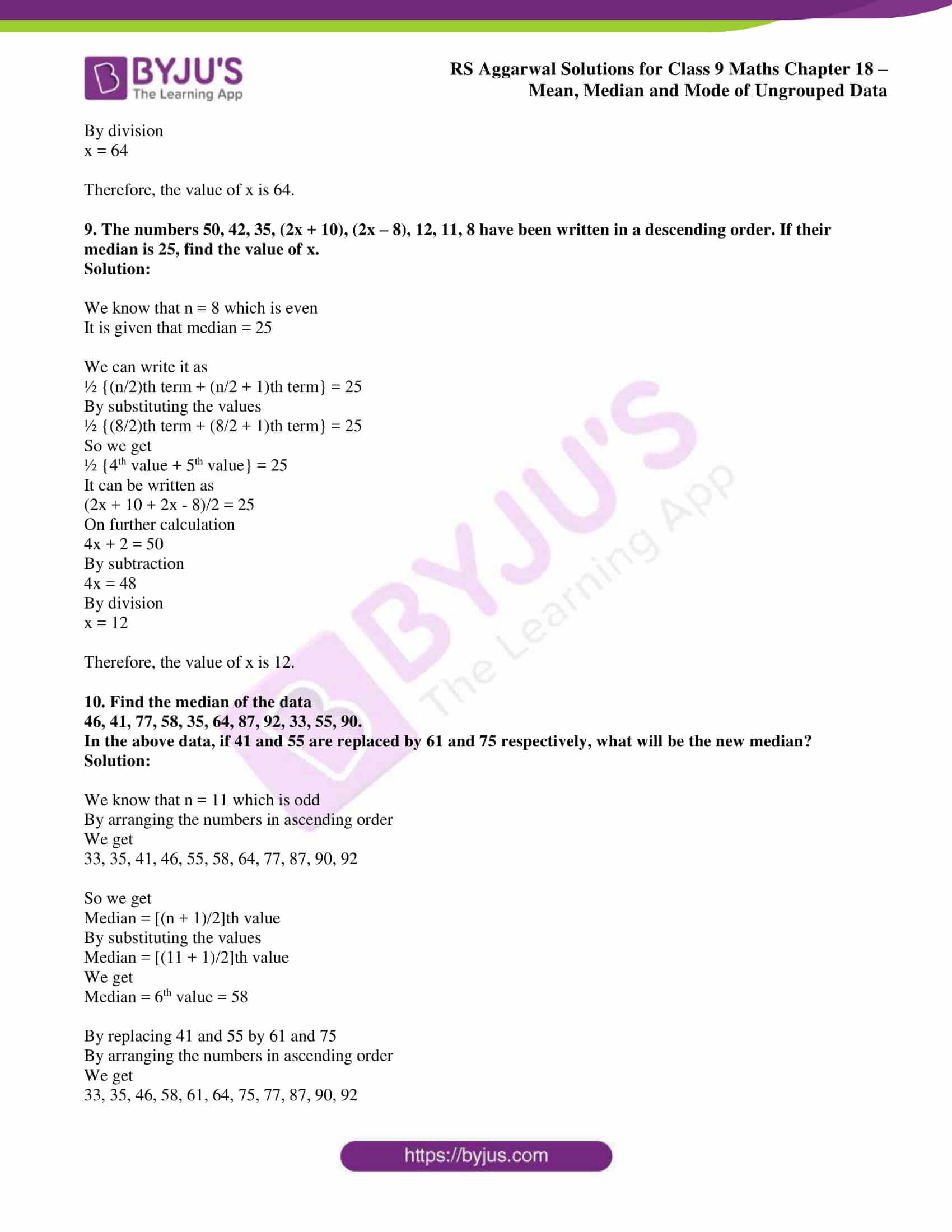## Access RS Aggarwal Solutions for Class 9 Chapter 18: Mean, Median and Mode of Ungrouped Data Exercise 18C

1. Find the median of:

(i) 2, 10, 9, 9, 5, 2, 3, 7, 11

(ii) 15, 6, 16, 8, 22, 21, 9, 18, 25

(iii) 20, 13, 18, 25, 6, 15, 21, 9, 16, 8, 22

(iv) 7, 4, 2, 5, 1, 4, 0, 10, 3, 8, 5, 9, 2

Solution:

(i) By arranging the numbers in ascending order

We get

2, 2, 3, 5, 7, 9, 9, 10, 11

We know that n = 9 is odd

So we get

Median = ½ (n + 1) th term

By substituting the values

Median = ½ (9 + 1) th term

It can be written as

Median = value of the 5th term = 7

We get

Median = 7

(ii) By arranging the numbers in ascending order

We get

6, 8, 9, 15, 16, 18, 21, 22, 25

We know that n = 9 is odd

So we get

Median = ½ (n + 1) th term

By substituting the values

Median = ½ (9 + 1) th term

It can be written as

Median = value of the 5th term = 16

We get

Median = 16

(iii) By arranging the numbers in ascending order

We get

6, 8, 9, 13, 15, 16, 18, 20, 21, 22, 25

We know that n = 11 is odd

So we get

Median = ½ (n + 1) th term

By substituting the values

Median = ½ (11 + 1) th term

It can be written as

Median = value of the 6th term = 16

We get

Median = 16

(iv) By arranging the numbers in ascending order

We get

0, 1, 2, 2, 3, 4, 4, 5, 5, 7, 8, 9, 10

We know that n = 13 is odd

So we get

Median = ½ (n + 1) th term

By substituting the values

Median = ½ (13 + 1) th term

It can be written as

Median = value of the 7th term = 4

We get

Median = 4

2. Find the median of:

(i) 17, 19, 32, 10, 22, 21, 9, 35

(ii) 72, 63, 29, 51, 35, 60, 55, 91, 85, 82

(iii) 10, 75, 3, 15, 9, 47, 12, 48, 4, 81, 17, 27

Solution:

(i) By arranging the numbers in ascending order

We get

9, 10, 17, 19, 21, 22, 32, 35

We know that n = 8 which is even

So we get

Median = ½ {(n/2)th term + (n/2 + 1)th term}

It can be written as

Median = ½ {4th term + 5th term)

By substituting the values

Median = ½ (19 + 21)

On further calculation

Median = ½ (40)

We get

Median = 20

(ii) By arranging the numbers in ascending order

We get

29, 35, 51, 55, 6, 63, 72, 82, 85, 91

We know that n = 10 which is even

So we get

Median = ½ {(n/2)th term + (n/2 + 1)th term}

It can be written as

Median = ½ {5th term + 6th term)

By substituting the values

Median = ½ (60 + 63)

On further calculation

Median = ½ (123)

We get

Median = 61.5

(iii) By arranging the numbers in ascending order

We get

3, 4, 9, 10, 12, 15, 17, 27, 47, 48, 75, 81

We know that n = 12 which is even

So we get

Median = ½ {(n/2)th term + (n/2 + 1)th term}

It can be written as

Median = ½ {6th term + 7th term)

By substituting the values

Median = ½ (15 + 17)

On further calculation

Median = ½ (32)

We get

Median = 16

3. The marks of 15 students in an examination are

25, 19, 17, 24, 23, 29, 31, 4, 19, 20, 22, 26, 17, 25, 21.

Find the median score.

Solution:

By arranging the numbers in ascending order

We get

17, 17, 19, 19, 20, 21, 22, 23, 24, 25, 26, 29, 31, 35, 40

We know that n = 15 which is odd

So we get

Median = ½ (n + 1) th term

By substituting the values

Median = ½ (15 + 1) th term

It can be written as

Median = value of the 8th term = 23

Therefore, the median score is 23.

4. The heights (in cm) of 9 students of a class are

148, 144, 152, 155, 160, 147, 150, 149, 145.

Find the median height.

Solution:

By arranging the numbers in ascending order

We get

144, 145, 147, 148, 149, 150, 152, 155, 160

We know that n = 9 which is odd

So we get

Median = [(n + 1)/2]th value

By substituting the values

Median = [(9 + 1)/2]th value

We get

Median = 5th value = 149cm

Therefore, the median height is 149cm.

5. The weights (in kg) of 8 children are

13.4, 10.6, 12.7, 17.2, 14.3, 15, 16.5, 9.8.

Find the median weight.

Solution:

By arranging the numbers in ascending order

We get

9.8, 1.6, 12.7, 13.4, 14.3, 15, 16.5, 17.2

We know that n = 8 which is even

So we get

Median = ½ {(n/2)th term + (n/2 + 1)th term}

It can be written as

Median = ½ {4th term + 5th term)

By substituting the values

Median = ½ (13.4 + 14.3)

On further calculation

Median = ½ (27.7)

We get

Median = 13.85kg

Therefore, the median weight is 13.85kg.

6. The ages (in years) of 10 teachers in a school are

32, 44, 53, 47, 37, 54, 34, 36, 40, 50.

Find the median age.

Solution:

By arranging the numbers in ascending order

We get

32, 34, 36, 37, 4, 44, 47, 50, 53, 54

We know that n = 10 which is even

So we get

Median = ½ {(n/2)th term + (n/2 + 1)th term}

It can be written as

Median = ½ {5th term + 6th term)

By substituting the values

Median = ½ (40 + 44)

On further calculation

Median = ½ (84)

We get

Median = 42

Therefore, the median age is 42 years.

7. If 10, 13, 15, 18, x + 1, x + 3, 30, 32, 35, 41 are ten observations in an ascending order with median 24, find the value of x.

Solution:

By arranging the numbers in ascending order

We get

10, 13, 15, 18, x + 1, x + 3, 30, 32, 35, 41

We know that n = 10 which is even

So we get

Median = ½ {(n/2)th term + (n/2 + 1)th term}

It can be written as

Median = ½ {5th term + 6th term)

By substituting the values

Median = ½ (x + 1 + x + 3)

On further calculation

Median = ½ (2x + 4)

We get

Median = x + 2

It is given that median = 24

So we get

x + 2 = 24

On further calculation

x = 24 – 2 = 22

Therefore, the value of x is 22.

8. The following observations are arranged in ascending order:

26, 29, 42, 53, x, x + 2, 70, 75, 82, 93.

If the median is 65, find the value of x.

Solution:

We know that n = 10 which is even

It is given that median = 65

We can write it as

½ {(n/2)th term + (n/2 + 1)th term} = 65

By substituting the values

½ {(10/2)th term + (10/2 + 1)th term} = 65

So we get

½ {5th value + 6th value} = 65

It can be written as

(x + x + 2)/2 = 65

On further calculation

2x + 2 = 130

By subtraction

2x = 128

By division

x = 64

Therefore, the value of x is 64.

9. The numbers 50, 42, 35, (2x + 10), (2x – 8), 12, 11, 8 have been written in a descending order. If their median is 25, find the value of x.

Solution:

We know that n = 8 which is even

It is given that median = 25

We can write it as

½ {(n/2)th term + (n/2 + 1)th term} = 25

By substituting the values

½ {(8/2)th term + (8/2 + 1)th term} = 25

So we get

½ {4th value + 5th value} = 25

It can be written as

(2x + 10 + 2x – 8)/2 = 25

On further calculation

4x + 2 = 50

By subtraction

4x = 48

By division

x = 12

Therefore, the value of x is 12.

10. Find the median of the data

46, 41, 77, 58, 35, 64, 87, 92, 33, 55, 90.

In the above data, if 41 and 55 are replaced by 61 and 75 respectively, what will be the new median?

Solution:

We know that n = 11 which is odd

By arranging the numbers in ascending order

We get

33, 35, 41, 46, 55, 58, 64, 77, 87, 90, 92

So we get

Median = [(n + 1)/2]th value

By substituting the values

Median = [(11 + 1)/2]th value

We get

Median = 6th value = 58

By replacing 41 and 55 by 61 and 75

By arranging the numbers in ascending order

We get

33, 35, 46, 58, 61, 64, 75, 77, 87, 90, 92

So we get

Median = [(n + 1)/2]th value

By substituting the values

Median = [(11 + 1)/2]th value

We get

Median = 6th value = 64

Therefore, the new median is 64.

### Access other exercise solutions of Class 9 Maths Chapter 18: Mean, Median and Mode of Ungrouped Data

Exercise 18A Solutions 31 Questions

Exercise 18B Solutions 14 Questions

Exercise 18D Solutions 8 Questions

### RS Aggarwal Solutions Class 9 Maths Chapter 18 – Mean, Median and Mode of Ungrouped Data Exercise 18C

RS Aggarwal Solutions Class 9 Maths Chapter 18 Mean, Median and Mode of Ungrouped Data Exercise 18C has answers to problems which help in finding the median of ungrouped data.

### Key features of RS Aggarwal Solutions for Class 9 Maths Chapter 18: Mean, Median and Mode of Ungrouped Data Exercise 18C

• The solutions are structured systematically based on the exam pattern and weightage of concepts.
• The students can improve problem solving and logical thinking abilities which are required to solve tricky problems.
• The problems in RS Aggarwal textbook are based on the latest CBSE syllabus and guidelines.
• Important formulas and various methods used in solving the problems are explained to improve conceptual knowledge among students.